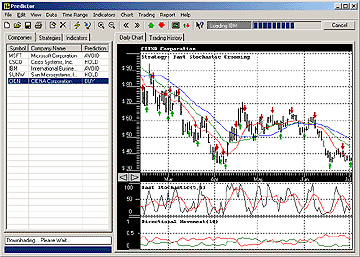# Williams %R Change Direction

This strategy is based on an absolute values of Williams %R. Typically when Williams %R reaches level of -20 it is considered that a security is overbought and when Williams %R is hitting -80 it is considered that a security is oversold. The current strategy does the following : it generates a buy signal when Williams %R is less than lower limit w (w = - 90 by default) and changing in an upward direction and generates sell signal when Williams %R is greater than the upper limit v (v = - 10 by default) and changing the direction downwards. Also a stop loss sell signal is included, it is triggered when the current closing price falls lower the last buying price minus %p (stop loss percentage)

### Formula

IF Williams(t-1) > Williams(t) AND
Williams(t-1) > v
THEN GO SHORT
ELSE
IF Williams(t-1) < Williams(t) AND
Williams(t-1) < w
THEN GO LONG
and v > w; e.g. v = -10,w = -90,
C(t) - closing price; p - percentage loss